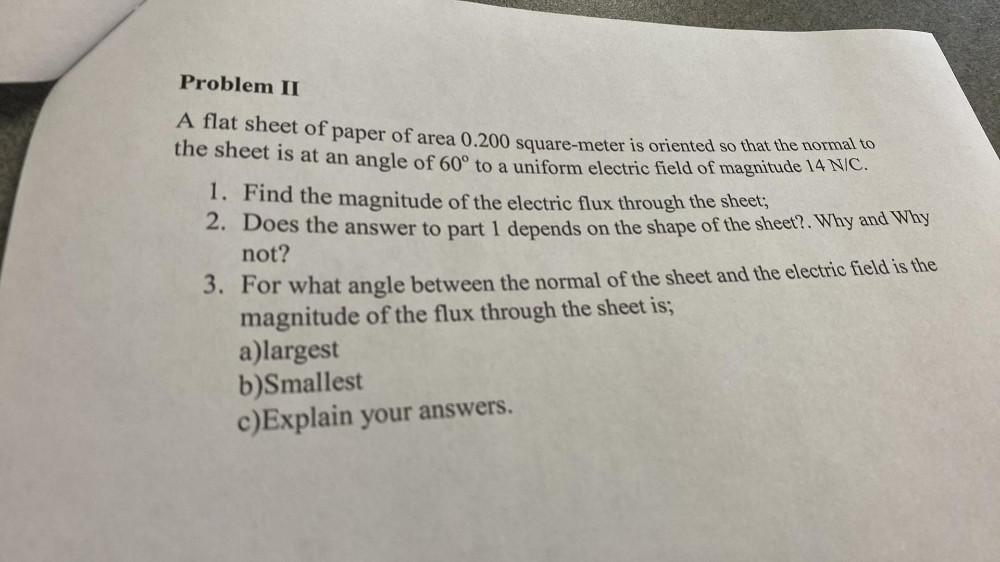Question:

# Problem II A flat sheet of paper of area 0.200 square meter is oriented so that the normal to the sheet is at an angle of 60° toProblem II A flat sheet of paper of area 0.200 square meter is oriented so that the normal to the sheet is at an angle of 60° to a uniform electric field of magnitude 14 NIC. 1. Find the magnitude of the electric flux through the sheet; 2. Does the answer to part 1 depends on the shape of the sheet?. Why and Why not? 3. For what angle between the normal of the sheet and the electric field is the magnitude of the flux through the sheet is; a)largest b)Smallest c)Explain your answers.